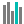#BETA.DIST (PQL - xl)

This function calculates the cumulative beta distribution function

• Library: PQL \ Spreadsheet \ Statistical
• Compatibility: Any content (regardless of data source) in the Tabulate spreadsheet module
• Solve: This function can be used with the Solve plug-in: both as part of the objective function and constraint functions

#### Syntax

BETA.DIST(x, alpha, beta, cumulative, OPTIONAL A, OPTIONAL B)

##### Function Arguments
 Name Description Type Optional x Value at which to evaluate the function, between the values of lower and upper Number alpha Alpha parameter of the distribution Number beta Beta parameter of the distribution Number cumulative A logical value that determines the function form. The function returns the cumulative distribution function if this argument is true. It returns the probability density function if the argument is false StringOrNumber A Lower bound of the interval for x; 0 if omitted Number Y B Upper bound of the interval for x; 1 if omitted Number Y